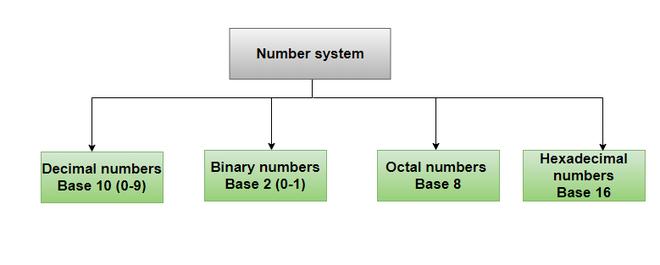Related Articles

# Is the Reciprocal of a Fraction always a Whole Number?

• Last Updated : 05 Aug, 2021

In order to represent numbers in a systematic way, a writing system was developed showing the integers in the proper manner known as the number system. The value of any digit can be easily obtained by obtaining its position in the number system and the base of the number system.

The number system most commonly used is the base 10 number system. However, it is not the only existing number system. The bases can exist of any number (from 2) and there will be a different pattern of explaining and placing digits for different bases. Base 2 is known as the Binary system and it is the language of the computer (only 0 and 1 are the digits known in this system), base 8 is known as the Octal system and base 16 is known as the Hexadecimal system.

Attention reader! All those who say programming isn't for kids, just haven't met the right mentors yet. Join the  Demo Class for First Step to Coding Coursespecifically designed for students of class 8 to 12.

The students will get to learn more about the world of programming in these free classes which will definitely help them in making a wise career choice in the future.### Fractions

Fractions are formed from the word “fractio” which means “to break”, hence, fractions are formed by breaking whole numbers. Fractions are a part of a whole number, a very good example of a fraction can be dividing a pizza among friends, imagine there are 5 friends and 1 pizza, so the pizza must be divided into 5 equal parts, therefore, every friend will get 1/5th of the pizza.

Reciprocal of a Fraction

The reciprocal of a fraction can be defined as the inverse of that fraction. Reciprocation, also known as multiplicative inverse, of any number, say x will be the Inverse of x, that is, 1/x or x-1. For example, the reciprocal of 5 is 1/5, the reciprocal of 3/2 is 2/3, and so on. In the easiest words, the numerator becomes the denominator and vice-versa.

Example 1: What is the reciprocal of 9/7?

The Reciprocal of 9/7 is 7/9.

Example 2: What is the reciprocal of 2? Is the reciprocal of 2 a whole number?

The reciprocal of 2 is 1/2. The reciprocal of 2 is not a whole number, 1/2 is a fraction.

### Is the Reciprocal of a Fraction always a Whole Number?

The reciprocal of a fraction is not always a whole number, it may be a fraction or whole number. The reciprocal of a fraction can have three cases,

• If the fraction is a proper fraction, the reciprocal of the fraction is an improper fraction. For example, the reciprocal of 2/5 is 5/2 which is an improper fraction.
• If the fraction is an improper fraction, the reciprocal of the fraction is a proper fraction. For example, the reciprocal of 7/3 is 3/7 which is a proper fraction.
• The reciprocal of a fraction is a whole number only when the numerator of the fraction is 1. For example, the reciprocal of 1/4 is 4 which is a whole number.

Example: Find the fractions whose reciprocals will give a whole number.

a. 1/5  b. 6/7  c. 1/9  d. 3/2